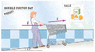# What formula do I use?

wr1015
What formula do I use?

A shopper pushes a 7.3 kg shopping cart up a 13° incline, as shown in Figure 5-21. Find the horizontal force, F, needed to give the cart an acceleration of 1.63 m/s2.

#### Attachments

•05-21.gif
18.6 KB · Views: 424

Homework Helper
What formula do I use?

Use $$\vec F = m \vec a$$

wr1015
Tide said:
Use $$\vec F = m \vec a$$

i did that and got 18.99 N but its being counted as wrong. There are some formulas in my book that show horizontal force as $$\sum\\F_{x}=F_{1},_{x}+F_{2},_{x}=F_{1}+F_{2}\cos\theta$$ but am not given any forces??Last edited: